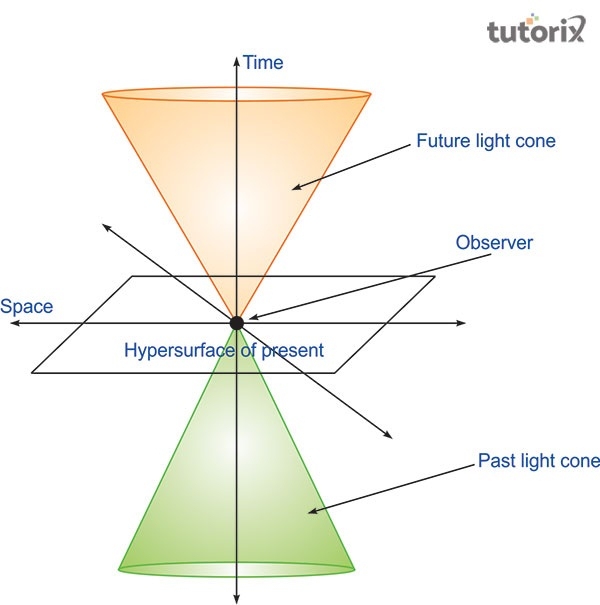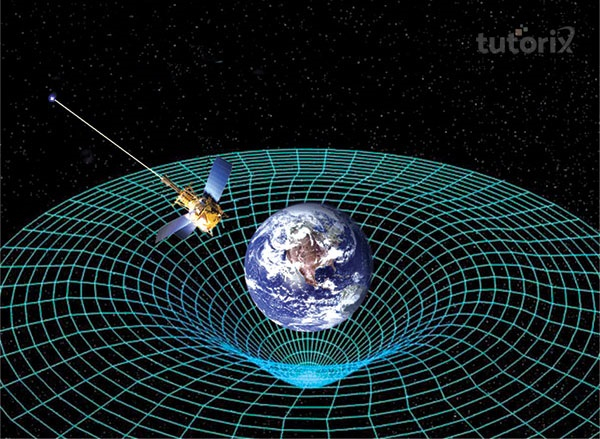# Relativity

## Introduction

Relativity is a notion that gives a statement that physics are the same everywhere. This theory is mainly related to time, space, and their relativity. Relativity is mainly defined that it is a state or quality of being relative. Relativity is a state of being dependent on the purpose of existence. This theory is related to gravity and the name of this theory mainly comes from the relativity of motion.

## What is Relativity?

It is a theory proposed by Einstein that describes the dependence of different physical phenomena on the observer’s relative motion. The observer reflects the behaviours and nature of time, space, gravity, and light (Nbcnews.com, 2022).

There are mainly two theories related to relativity and they are- the Theory of Special Relativity and the Theory of General Relativity.

Accordion g to Einstein, the laws of physics remains the same for all non-accelerating observers. It also shows that when light travels through a vacuum the speed remains the same and the speed of the observer does not matter from which it travels.

The two theories describe two different facts or relativity. In the Special Theory of Relativity, it is described that time is relative. On the other hand, the General theory says that planets do the same thing to space-time. This is the cause for the falling of all things towards the grounds.

## Example of Relativity

Relativity is a great concept related to space, time, and motion. An example of this factor of relativity can be given. Suppose on a train two people are playing ping-pong and the train is moving toward the North and the speed is around 30 m/s (Ducksters.com, 2022). When the two players hit the ball, it will move towards the North at a speed of 2 m/s. It will also travel at a speed of 2 m/s toward the south. The observers can always see from the side of the train, as it is moving towards the north always.

## Theory of Special Relativity

The Theory of Special Relativity by Albert Einstein is nothing but an explanation of the matter that a change within an object mainly affects measurements of mass, space, and time. Experiments on electromagnetism say that an electromagnetic field wave can zip through empty space (Sciencealert.com, 2022).

Speed can affect space and time greatly and the Third Law of Motion says the matter. This law describes the relationship of an object’s acceleration and mass to the force.Figure 1: Theory of special relativity

The solution to this theory goes to the factor that both space and time is relative factors. According to this theory, time and distance affect the change in acceleration (Kontou & Sanders, 2020).

Einstein shows that when an object is at rest the total mass of the object is equal to its energy time. It is seen when the light’s speed is constant then energy and mass seem to be the same.

## Theory of General Relativity

General Relativity is a theory of gravitation made by Albert Einstein. According to this theory, the gravitational effect that is observed between the masses gives a result of their warping of space-time (Ishak, 2019).

This theory of gravity gives the basic idea that gravity is a curving of space. As per the theory when an object becomes more massive, it wraps more space around it. Theory of General Relativity is mainly used for predicting the existence of black holes.Figure 2: Theory of General Relativity

This theory is a useful theory that decides the bending of light due to gravity. The behaviours of planets on their orbits are also defined through this theory. This theory is named in this way because it includes gravity.

## Theory of Relativity in Real Life

The Theory of Relativity explains the behaviours of objects in time and space. It is used in the existence of black holes, the behaviour of planets on objects, bending of lights due to gravity (Compère, 2019).

The rates of satellite clocks are adjusted with this concept. Mathematical corrections are made using those theories in daily life. These are the use of relativity theory in the daily life of humans.

## Conclusion

Relativity is a theory of Einstein that includes the theory of gravity. The main factors of this theory are related to space, time, motion, and mass. These are the important aspect of this relativity. It includes two types of theory -the Special Theory and the General Theory of Relativity.

The different uses of relativity in the daily life of people say its importance in the living world. On the other hand, the Theory of Special relativity explains how speed affects space, time, and mass.

## FAQs

Q1. What are the main principles of general relativity?

ans. There are two main principles of general relativity. The principle says that the theory of special relativity helps govern the local physics. The equivalence principle says that it is tough for an observer to distinguish between acceleration and gravity.

Q2. What is general relativity used for?

Ans. General Relativity is a theory related to gravity and it is used for explaining the behaviours of objects in time and space. It is also used for the prediction of things like the existence of black holes, the behaviour of planets, and deciding of light bending due to gravity.

Q3. What is the daily life application of relativity?

Ans. Laser devices, GPS Navigators, The Physics of financial markets and Photoelectric cells are the daily use of relativity in the everyday life. Einstein develops the theory of relativity for making easy the life of people through it.

Q4. What was the concept of Einstein about time?

Ans. According to Einstein the Special Theory of Relativity describes the matter that time is relative. Differently, it can say that time passes at a rate that depends on the frame of reference.